# Ground Plane Segmentation of Lidar Data on FPGA

This example shows how to separate organized 3-D lidar point cloud data into ground and non-ground parts on FPGA. Ground plane removal is an essential preprocessing step in lidar applications.

The example model loads a Velodyne PCAP® file from HDL-32E Lidar sensor. The PointCloudReader subsystem uses readFrame to read the input lidar data and separate its location and intensity components. The SegmentGroundFromLidarDataHDL subsystem performs ground plane segmentation in two major steps, Savitsky - Golay smoothing and breadth-first search ground labeling. The PointCloudViewer subsystem creates a point cloud player using pcplayer to observe the segmented lidar data. The lidar must be mounted horizontally such that all ground points are observed in the lidar scan closest to the sensor. For more details, see segmentGroundFromLidarData (Computer Vision Toolbox).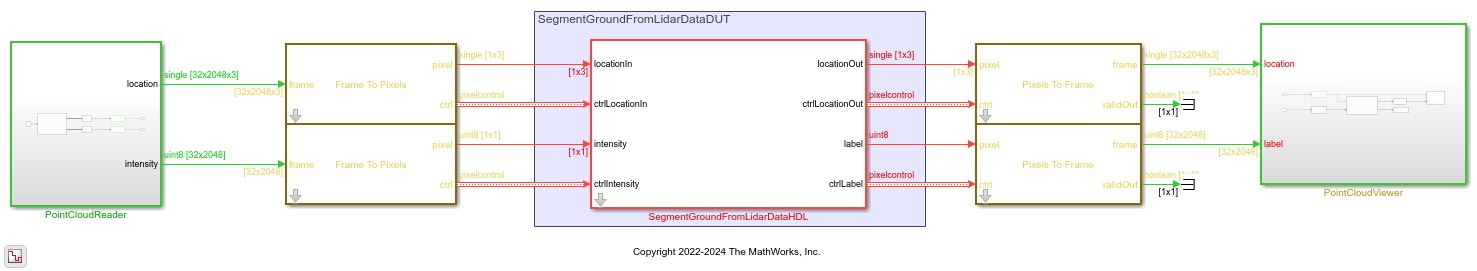### Ground Segmentation HDL Subsystem

The input to the HDL subsystem is an organized point cloud, available as a 32-by-2048-by-3 array. The three channels represent the x-, y-, and z- coordinates of the points. The CartesianToSphericalProjection converts these coordinates into a spherical system of range, pitch, and yaw. The SatizskyGolaySmoothing subsystem interpolates any non-finite outlier values in the lidar measurements. Finally, the BFSGroundLabeling subsystem flood fills the space and labels the connected ground components. To reduce latency and resource use in the flood fill operation, the model reads the values in an upside-down (row-wise) order.### Savitzky Golay Interpolate Subsystem

SavitzkyGolayInterpolate subsystem interpolates the non-finite values in the range and pitch plane. For the range plane, the interpolation is done using the neighbors in the column. If the difference of the neighbors and the current pixel is less than the required rangeThreshold, the mean of the differences is the new interpolated value of the current non-finite pixel. For the pitch plane, the interpolation of the current non-finite pixel is done using the left neighbor in the row. Using only the left neighbor optimizes the resources of the design while keeping up with the performance.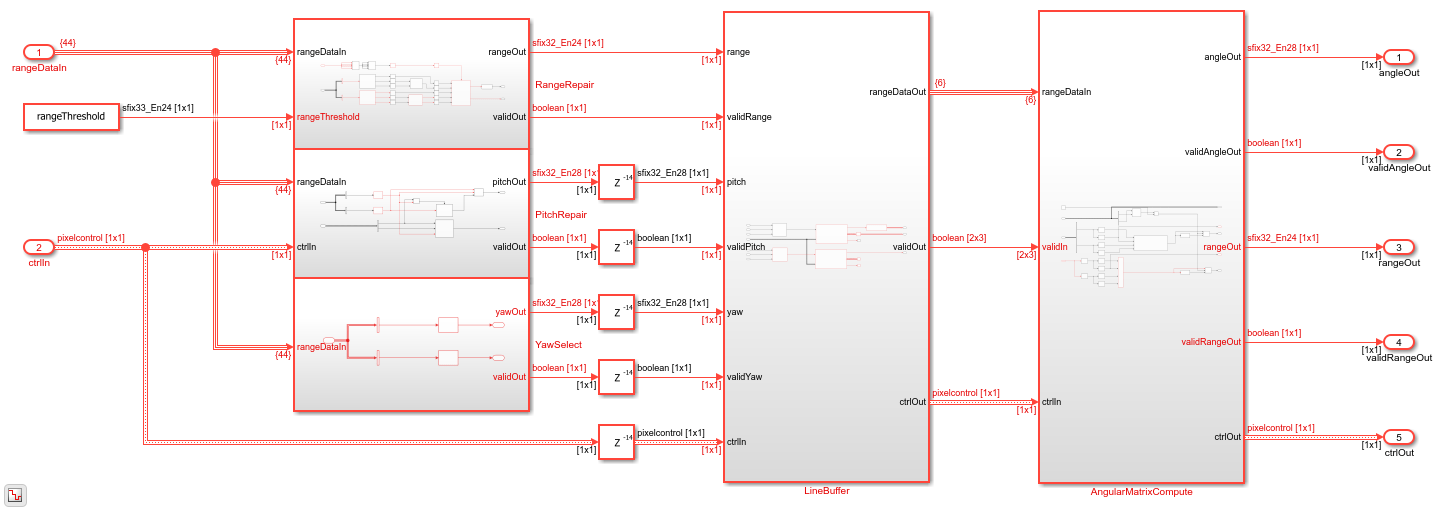### Angle Compute Subsystem

After interpolation, the AngleCompute subsystem computes the angle of inclination derived from consecutive range values. It takes each column of the range image and calculates the angle. The FloodFill subsystem uses this angle to threshold and label the ground points. Let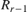andbe the range values corresponding to the rowsandin the range image, then the angle can be computed as,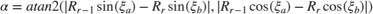. Here,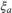and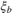are the vertical angles or pitch values corresponding to the rowsand, respectively.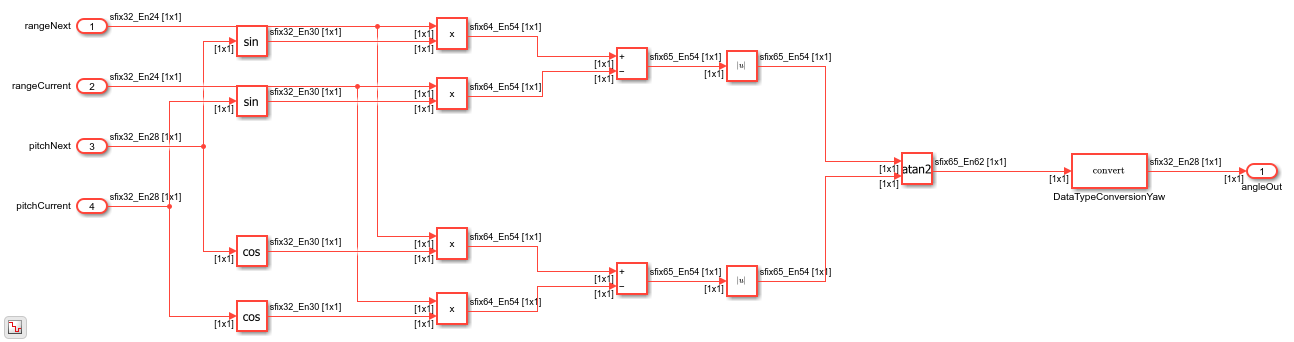### Flood Fill Subsystem

The BFSGroundLabeling subsystem uses the flood fill algorithm to segment the ground points. It involves two steps implemented by the subsystems SeedsCompute and FloodFill. The seeds act as a start point for segmenting the ground points. The seedThreshold is the initial elevation angle used to identify the ground point in the scanning line closest to the lidar sensor. SeedsCompute subsystem checks if the elevation angle falls below the threshold and marks valid seeds as ground points for every column.

The FloodFill subsystem labels points as ground and non-ground points. The flood fill algorithm accuracy increases with more iterations. However, each iteration requires more hardware resources. This example implements a single iteration that filters more than 90% of the points accurately. The FloodFill subsystem computes the elevation angle difference between one labeled ground point and its 4-connected neighbors, i.e., top, bottom, left, and right. If any of the four neighbors is a ground pixel and the angle difference is less than a specified angleThreshold, then the point is labeled as ground point. The GroundLabelCompute subsystem is shown below.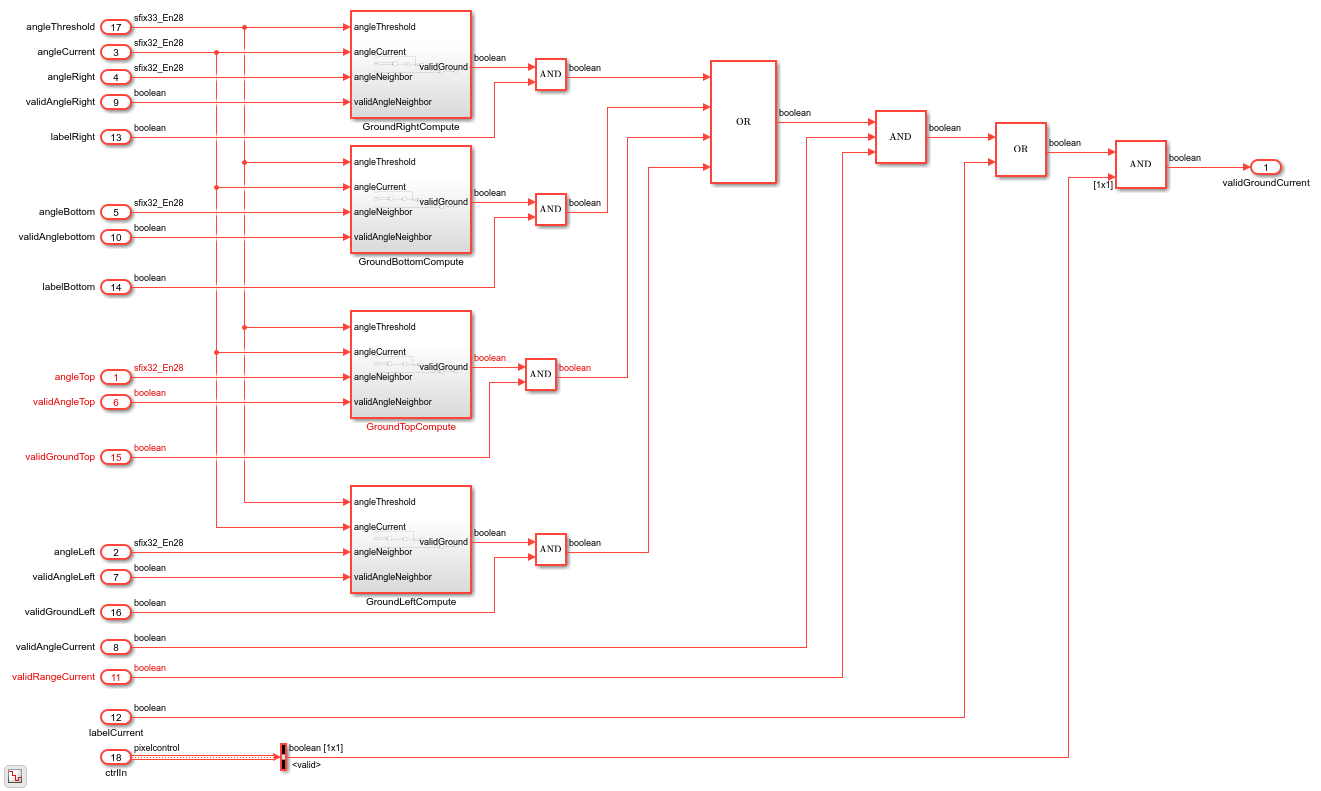### Simulation and Output

The PointCloudViewer subsystem uses the ground point labels from the point cloud to color all ground points green and non-ground points white and plot the resulting lidar point cloud. The figure below shows the result of ground plane segmentation for HDL-32E lidar sensor.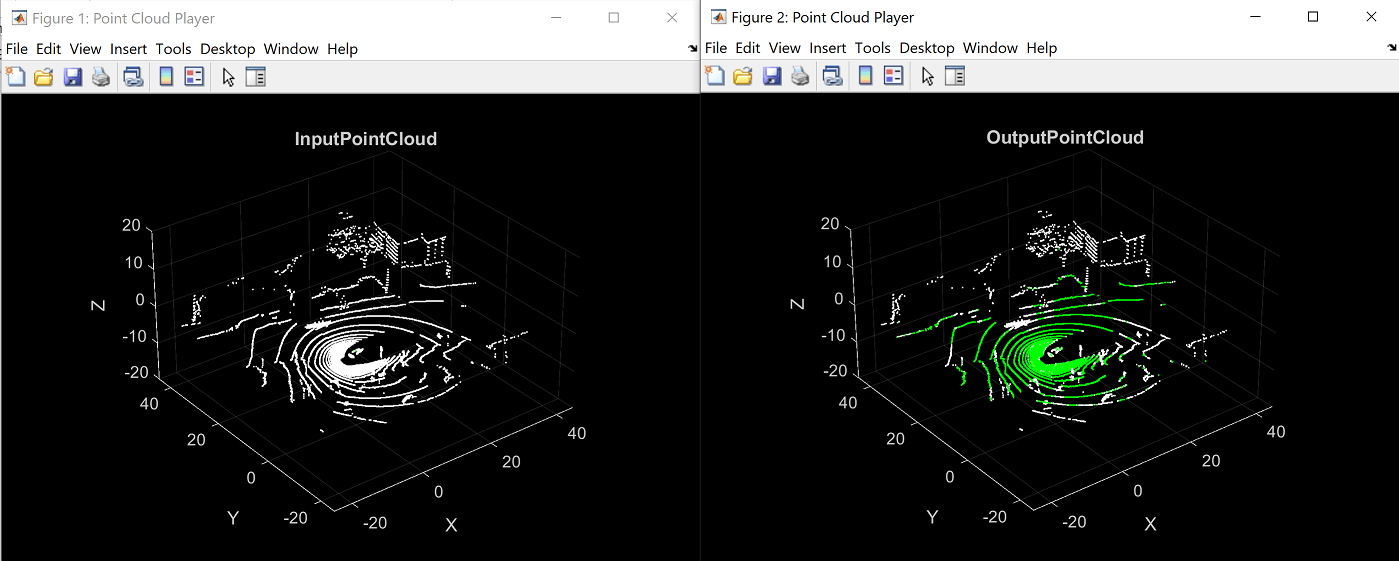### Implementation Results

To check and generate the HDL code referenced in this example, you must have the HDL Coder™ product. To generate the HDL code, use this command.

makehdl('SegmentGroundFromLidarDataHDL/SegmentGroundFromLidarDataHDL') 

The generated code was synthesized for a target of Xilinx ZC706 SoC. The design met a 150 MHz timing constraint. The hardware resources are as shown in the table.

T = 5x2 table Resource Usage ________ ______________ DSP48 26 (2.89%) Register 39035 (8.93%) LUT 33550 (15.35%) BRAM 160 (29.36%) URAM 0 (0%) 

### References

 Bogoslavskyi, I. "Efficient Online Segmentation for Sparse 3D Laser Scans." Journal of Photogrammetry, Remote Sensing and Geoinformation Science. Vol. 85, Number 1, 2017, pp. 41-52.